# Where's my cheese?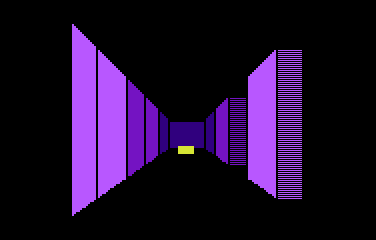This is a little game for the 2016 NOMAM's BASIC 10-liners Contest. This program fits in the EXTREM-256 category, and it was written using TurboBASIC XL 1.5 for the 8-bits ATARI XL/XE. Development started on 2015-08-29, and it took 3+7 days. The final version's date is 2016-02-17.

UPDATE: It obtained the 1st place of 20 entries in the category.

## Description

You are a hungry lab mouse. Run through the maze to find and enjoy a slice of cheese.

## Instructions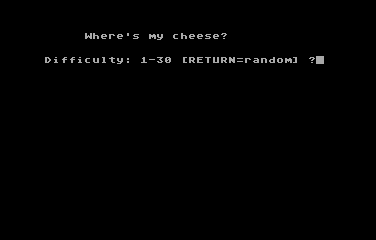Select the desired level. It's related with the size of the maze. Level 30 has 961 cells to walk through.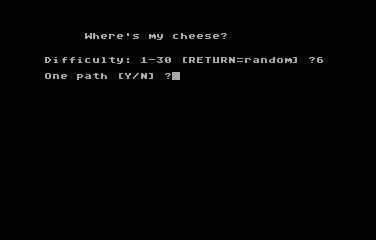Choose if you want only a single path to solve the maze. Extra paths could help you to find the cheese or it might create loops in your way.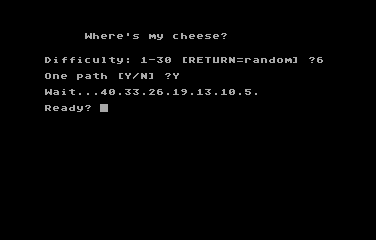Wait for a moment while the maze is being created, then pres a key to start.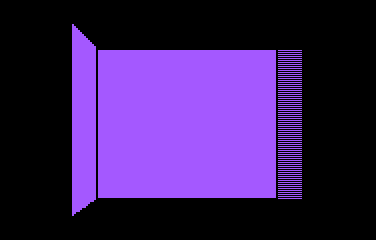If you are in front of a wall, you must turn left or right. You can turn by moving the joystick to the sides. Moving the joystick up, you go foward. You cannot step back without rotating in 180°.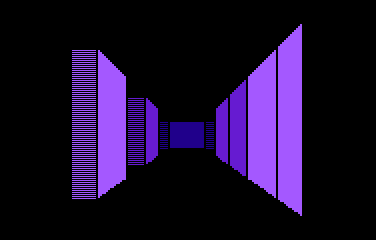Trust your instincts and choose the way you prefer.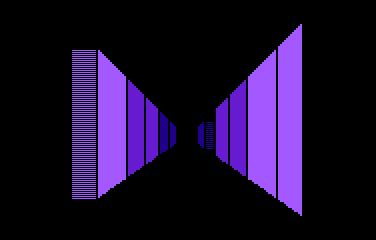If there is a long path in front of you, you'll see nothing but a dark zone.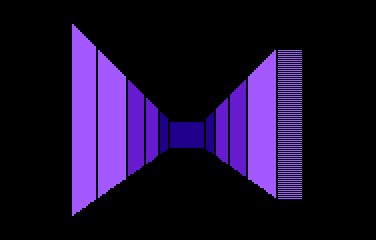Sometimes you may find dead-ends and have to turn around and walk back.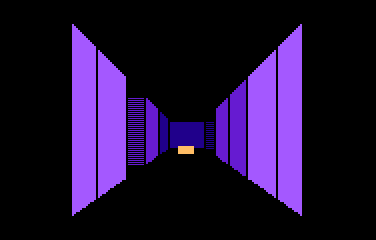If you are lucky, you may find the cheese.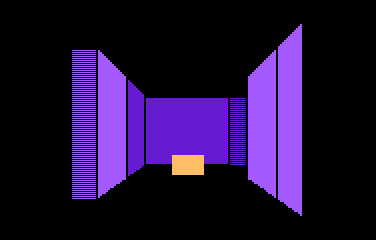Go and eat that delicious piece of cheese.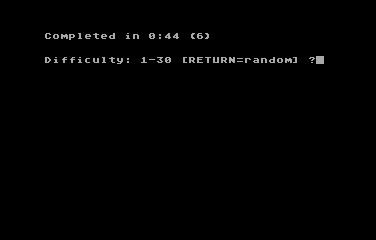When you get the cheese, the total time taken for the search is displayed. You can select a new difficulty level and start another search.

## Development of the game

Soon!

Get the CHEESE.ATR file and set it as drive 1 in a real Atari or emulator. Turn on the computer and the game should start after loading. A joystick in port 1 is required.

## The code

The abbreviated BASIC code is the following: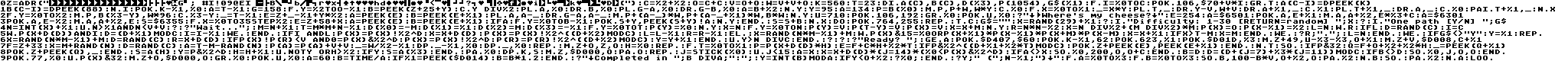The full and expanded BASIC listing is:

 ``` z=adr("binary data") ``` Losts of binary data: 1-8 (8 bytes): Horizontal position of players and missiles. These are R/W bytes... this means that the program listing will change if listed after a run. 9-16 (8 bytes): Default horizontal position for each P/M. These are copied into the previous group on each iteration of the game. 17-21 (5 bytes): Width of every player (4 bytes) and missiles (1 byte, 2 bits each). 22-27 (6 bytes): 3 pairs of coordinates and height of the front wall for each screen. 28-33 (6 bytes): length of the projection of the walls. 34-49 (16 bytes): 8 pairs of P/M index and horizontal position. 50-58 (9 bytes): Color for all P/M, playfield and background. 59-94 (36 bytes): 18 pairs of value and number of repetitions to fill P/M area. ```c=%2+%2 o=c+c v=o+o w=v+v+o k=560 t=23 ``` Constants for frequently used numbers: C=4:O=8:V=16:W=40. All of them, as well as %0 to %3 constants, save space in a tokenized line of code, so longer lines could be entered. ```dim a(c),b(c),d(%3),p(1054),g\$(%1) ``` Arrays: A(0-4): SDLIST vector for each captured screen (DPEEK(560)). B(0-4): SAVMSC vector for each captured screen (DPEEK(88)). P(0-1054): Array that stores the connection paths of the maze between cells, and also store the cheese position. D(0-3): Array that stores the amount of cells required to move in a given direction: 0=N, 1=E, 2=S, 3=W. G\$: Values specified by the player to alow extra paths creation. ```for i=%0 to c ``` Routine to set up the vectors for the 4 cached screens plus 1 extra screen that is used to initialize RAM where P/M data will be stored: ``` poke 106,\$70+v*i ``` Sets a custom RAMTOP register for each screen. Each screen requires 4K as explained below, so the pointer is moved 16 memory pages each time. ``` graphics t ``` Creates the screen at that given address. Each of them is a graphics mode 7+16 screen: 160x96 pixels of 4 colours and no text box. Every screen uses 3840 bytes of data plus 200 bytes for the display list, so we have enougth space in 4K. ``` a(c-i)=dpeek(k) b(c-i)=dpeek(88) ``` Capture the DL (SDLIST) and screen data (SAVMSC) vectors. ```next i ``` End of the vectors capture routine. ```poke k-%1,%0 ``` Turns of the screen (DMA). ```a=t-%1 g=158 for y=%2 to o-%1 b=peek(z+25+y) color y div%2 plot a,%0 drawto b,%0 plot g-a,%0 drawto g-b,%0 a=b+%2 next y ``` Draws a line with different colors and proportional lengths, with a black dot between them and the shortest segments in the center. Each segment length was stored in the binary data string. ```p=b(%0) move p,p+w,w*y ``` Copies the first line into the other lines of the screen to create vertical columns of solid color. ```y=95 a=134 color %0 for x=%0 to %1 _=x*y plot t,_ drawto y-v,w+v drawto a+%1,_ color %1 plot t+%1,_ drawto a,_ color %0 paint t+%1,_ next x ``` Creates a vanishing point clearing out triangles for the ceiling and the floor. The code without the loop and explicit constant values is: ```color 0 (cont) plot t,0 plot t,95 drawto 79,56 drawto 79,56 drawto 23,0 drawto 23,95 color 1 color 1 plot 23,0 plot 23,95 drawto 134,0 drawto 134,95 color 0 color 0 paint 22,0 paint 22,95 ``` ```for y=%0 to %2 move p,b(%3-y),w*96 color %3-y _=t-%1 e=z+_-%1+y*%2 a=peek(e) b=peek(e+%1) plot a,a-_ drawto g-a,a-_ move p+(a-_)*w,p+(a-_+%1)*w,b*w next y ``` ```for y=0 to 2 move p,b(3-y),3840 color 3-y e=z+21+y*2 a=peek(e) b=peek(e+1) plot a,a-22 drawto 158-a,a-22 move p+(a-22)*40,p+(a-21)*40,b*40 next y ``` Saves current image with a long passage as screen 3, then draws a far wall and saves as screen 2, a middle wall as 1, and leaves nearest wall as current screen 0. Position and size of every wall is read from binary data. ```u=710 ``` Constant to save bytes on some lines. This is the text background color register for graphics mode 0. ```poke 106,192 graphics %0 poke u,%0 ? "Where's my cheese?" ``` Restore RAMTOP to the default value and creates a black text screen to request for game parameters. ```e=254 a=\$6501 poke a,e+%1 move a,a+%2,e*%3+c a=\$6301 poke a,e-%2 move a,a+%2,e s=\$6355 for x=%0 to 35 step %2 e=z+58+x a=peek(e) b=peek(e+%1) if a for y=%0 to b-%1 poke s+y,peek(s+y)!a next y endif s=s+b next x ``` Set up P/M data reading patterns from binary string. ```do ``` Main loop... ``` poke 764,255 ``` Clears the keypress register to avoid unwanted parameters. ``` repeat trap c g\$="" x=rand(29)+%1 ? input "Difficulty: 1-30 [RETURN=random] ";x ? input "One path [Y/N] ";g\$ n=int(x)+%1 until n>%1 and nt-m x=m endif wend ? r;"."; l=n endif ``` If there was not an available cell adjacent to the current one, or the number of cells assigned to a path in a single run was reached, it's time to find another cell already assigned that has an adjacent unassigned cell. This resets the single run length. ``` wend ``` Loop until the maze is complete. ``` if g\$<>"Y" y=%1 repeat x=rand(n*m-%1)+m d=rand(c) r=x+d(d) if p(x)!p(r)n div c endif ``` If it was selected by the player, add extra paths randomly in the maze. ``` ? ? ? "Ready? "; get a ``` Waits for a key to begin. ``` poke \$D407,\$60 poke k-%1,62 poke 623,%1 poke \$D01D,%3 ``` Prepare P/M graphics. ``` move z+49,u-%3-%3,o+%1 ``` Set up the colors of every player and missile. ``` move z+v,\$D008,c+%1 ``` Set the position and width of every player and missile. ``` f=z+33 ``` Pointer to the P/M horizontal positions table for overlays. ``` x=m+rand(n) d=rand(c) ``` Assigns the player a random starting point in the north bound and puts him looking at a random direction. ``` a=t-m-rand(n) p(a)=p(a)+v+v ``` Assigns a random place to hide the cheese in the south bound of the maze. ``` _=w/%2-%1 dpoke _-%1,%0 dpoke _,%0 ``` Reset the internal timer. ``` repeat ``` Game loop... ``` move z+o,z,o ``` Prepares the overlays for the next screen to display. ``` h=%0 ``` H is the number of steps that could be walked straight forward without hitting a wall, with a maximum of 3 steps. ``` repeat ``` This routine builds the screen based on where the player is and in what direction is he looking. Starts from the cell where the player is and advances one step on every loop. ``` for t=%0 to %1 ``` Checks if there is an open path to the sides, first to the right, then to the left. ``` p=p(x+d(d)*h) ``` Gets the cell to be analyzed. ``` e=f+c*h+%2*t ``` Finds the overlay P/M pointers for current step. ``` if p&%2^((d+%1+%2*t) mod c) poke z+peek(e),peek(e+%1) endif ``` If there is an open path to the checked side, enables the overlay to display that path in the screen. ``` next t ``` Now, the other side for this position... ``` sound ``` Turns off any sound that has been played. ``` if p&32 q=f+o+%2+%2*h _=peek(q+%1) poke z+peek(q),_ endif ``` Checks if the analyzed cell has the cheese. If the cheese is there, finds the P/M pointer to select the player or missile that must be displayed. Note that there are only 2 positions where the cheese is displayed. It won't be neither in the player's cell (H=0) nor the darker zone (H>2). The underscore variable just allows the splitting of the POKE between two lines of the source code to save space. ``` s=a(h) ``` Sets the screen with front wall for the currently analyzed cell. ``` y=p&%2^d ``` Checks if there is a path to the front in the analyzed cell. ``` h=h+%1 ``` Sets the next cell in the forward direction to analyze... ``` until not y or h>%2 ``` ... unless there was a wall or that cell is in the darker zone (3 steps away). ``` if y s=a(%3) endif ``` If there was a path in the last check, then the darker zone must be displayed. ``` pause %0 ``` Synchronizes with vertical blank (VB) to minimize glitches. ``` dpoke k,s move z,\$D000,o ``` Changes to the new screen and assigns a new horizontal position for all P/M graphics at the same time to apply overlays to the screen. ``` pause o ``` Waits for a little moment before the check for the next movement. ``` repeat j=stick(%0) until j<15 ``` Waits until the joystick is moved. ``` a=x x=x+d(d)*(j=14)*(%0x sound %0,200,o,o+c endif ``` If the position changed, plays a walking sound. ``` b=d d=(d+(j=7)+%3*(j=11)) mod c ``` Save current direction and calculates the new one based on the joystick position. ``` if b<>d sound %0,j,o,o endif ``` If turned left or right, plays a sound. The pitch depends to where the turn was. ``` poke 77,%0 ``` Reset the attract mode counter to prevent screen color changes during the play. ``` until p(x)&32 ``` Ends the loop if the cheese was taken. ``` move z+o,\$D000,o ``` Moves all P/M out of the screen. ``` graphics %0 poke u,%0 ``` Returns to a black graphics 0 screen. ``` a=60 b=time/a if %1=peek(\$D014) b=b*1.2 endif ``` Calculates the time taken to find the cheese, and applies a correction factor for NTSC. ``` ? "Completed in ";b div a;":"; y=int(b) mod a if y